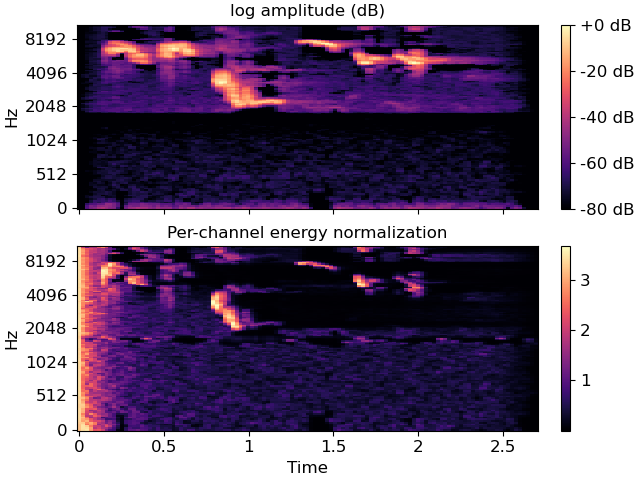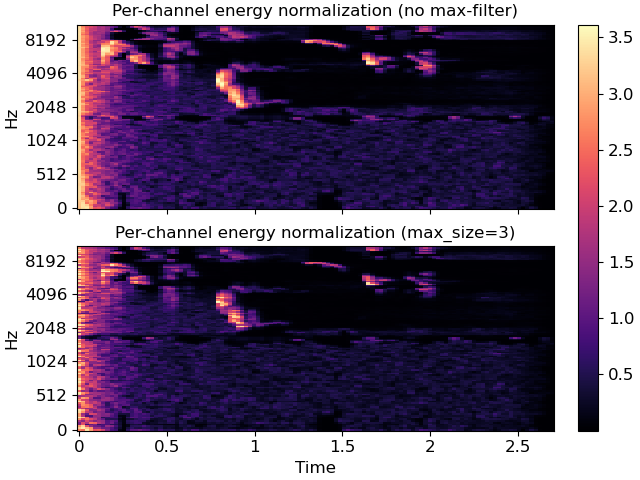# librosa.pcen¶

librosa.pcen(S, sr=22050, hop_length=512, gain=0.98, bias=2, power=0.5, time_constant=0.4, eps=1e-06, b=None, max_size=1, ref=None, axis=- 1, max_axis=None, zi=None, return_zf=False)[source]

Per-channel energy normalization (PCEN)

This function normalizes a time-frequency representation `S` by performing automatic gain control, followed by nonlinear compression 1

```P[f, t] = (S / (eps + M[f, t])**gain + bias)**power - bias**power
```

IMPORTANT: the default values of eps, gain, bias, and power match the original publication, in which `S` is a 40-band mel-frequency spectrogram with 25 ms windowing, 10 ms frame shift, and raw audio values in the interval [-2**31; 2**31-1[. If you use these default values, we recommend to make sure that the raw audio is properly scaled to this interval, and not to [-1, 1[ as is most often the case.

The matrix `M` is the result of applying a low-pass, temporal IIR filter to `S`:

```M[f, t] = (1 - b) * M[f, t - 1] + b * S[f, t]
```

If `b` is not provided, it is calculated as:

```b = (sqrt(1 + 4* T**2) - 1) / (2 * T**2)
```

where `T = time_constant * sr / hop_length`. 2

This normalization is designed to suppress background noise and emphasize foreground signals, and can be used as an alternative to decibel scaling (`amplitude_to_db`).

This implementation also supports smoothing across frequency bins by specifying `max_size > 1`. If this option is used, the filtered spectrogram `M` is computed as:

```M[f, t] = (1 - b) * M[f, t - 1] + b * R[f, t]
```

where `R` has been max-filtered along the frequency axis, similar to the SuperFlux algorithm implemented in `onset.onset_strength`:

```R[f, t] = max(S[f - max_size//2: f + max_size//2, t])
```

This can be used to perform automatic gain control on signals that cross or span multiple frequency bans, which may be desirable for spectrograms with high frequency resolution.

1

Wang, Y., Getreuer, P., Hughes, T., Lyon, R. F., & Saurous, R. A. (2017, March). Trainable frontend for robust and far-field keyword spotting. In Acoustics, Speech and Signal Processing (ICASSP), 2017 IEEE International Conference on (pp. 5670-5674). IEEE.

2

Lostanlen, V., Salamon, J., McFee, B., Cartwright, M., Farnsworth, A., Kelling, S., and Bello, J. P. Per-Channel Energy Normalization: Why and How. IEEE Signal Processing Letters, 26(1), 39-43.

Parameters
Snp.ndarray (non-negative)

The input (magnitude) spectrogram

srnumber > 0 [scalar]

The audio sampling rate

hop_lengthint > 0 [scalar]

The hop length of `S`, expressed in samples

gainnumber >= 0 [scalar]

The gain factor. Typical values should be slightly less than 1.

biasnumber >= 0 [scalar]

The bias point of the nonlinear compression (default: 2)

powernumber >= 0 [scalar]

The compression exponent. Typical values should be between 0 and 0.5. Smaller values of `power` result in stronger compression. At the limit `power=0`, polynomial compression becomes logarithmic.

time_constantnumber > 0 [scalar]

The time constant for IIR filtering, measured in seconds.

epsnumber > 0 [scalar]

A small constant used to ensure numerical stability of the filter.

bnumber in [0, 1] [scalar]

The filter coefficient for the low-pass filter. If not provided, it will be inferred from `time_constant`.

max_sizeint > 0 [scalar]

The width of the max filter applied to the frequency axis. If left as 1, no filtering is performed.

refNone or np.ndarray (shape=S.shape)

An optional pre-computed reference spectrum (`R` in the above). If not provided it will be computed from `S`.

axisint [scalar]

The (time) axis of the input spectrogram.

max_axisNone or int [scalar]

The frequency axis of the input spectrogram. If None, and `S` is two-dimensional, it will be inferred as the opposite from `axis`. If `S` is not two-dimensional, and `max_size > 1`, an error will be raised.

zinp.ndarray

The initial filter delay values.

This may be the `zf` (final delay values) of a previous call to `pcen`, or computed by `scipy.signal.lfilter_zi`.

return_zfbool

If `True`, return the final filter delay values along with the PCEN output `P`. This is primarily useful in streaming contexts, where the final state of one block of processing should be used to initialize the next block.

If `False` (default) only the PCEN values `P` are returned.

Returns
Pnp.ndarray, non-negative [shape=(n, m)]

The per-channel energy normalized version of `S`.

zfnp.ndarray (optional)

The final filter delay values. Only returned if `return_zf=True`.

Examples

Compare PCEN to log amplitude (dB) scaling on Mel spectra

```>>> import matplotlib.pyplot as plt
>>> y, sr = librosa.load(librosa.ex('trumpet'), duration=3)
```
```>>> # We recommend scaling y to the range [-2**31, 2**31[ before applying
>>> # PCEN's default parameters. Furthermore, we use power=1 to get a
>>> # magnitude spectrum instead of a power spectrum.
>>> S = librosa.feature.melspectrogram(y, sr=sr, power=1)
>>> log_S = librosa.amplitude_to_db(S, ref=np.max)
>>> pcen_S = librosa.pcen(S * (2**31))
>>> fig, ax = plt.subplots(nrows=2, sharex=True, sharey=True)
>>> img = librosa.display.specshow(log_S, x_axis='time', y_axis='mel', ax=ax)
>>> ax.set(title='log amplitude (dB)', xlabel=None)
>>> ax.label_outer()
>>> imgpcen = librosa.display.specshow(pcen_S, x_axis='time', y_axis='mel', ax=ax)
>>> ax.set(title='Per-channel energy normalization')
>>> fig.colorbar(img, ax=ax, format="%+2.0f dB")
>>> fig.colorbar(imgpcen, ax=ax)
```

Compare PCEN with and without max-filtering

```>>> pcen_max = librosa.pcen(S * (2**31), max_size=3)
>>> fig, ax = plt.subplots(nrows=2, sharex=True, sharey=True)
>>> librosa.display.specshow(pcen_S, x_axis='time', y_axis='mel', ax=ax)
>>> ax.set(title='Per-channel energy normalization (no max-filter)')
>>> ax.label_outer()
>>> img = librosa.display.specshow(pcen_max, x_axis='time', y_axis='mel', ax=ax)
>>> ax.set(title='Per-channel energy normalization (max_size=3)')
>>> fig.colorbar(img, ax=ax)
```